### 5Samplers and Solvers

The point of writing a generate model is typically not to simply run it to generate data, but rather to do inference on it—for example, to estimate parameters given observed data. This requires wrapping the model in a sampler or solver of some sort.

A sampler is an object that contains a probabilistic program and produces samples from the posterior distribution of its result expression. Samplers can be either unweighted (where each sample is equally representative of the posterior distribution) or weighted (where each sample comes with a factor that corrects for the ratio of its sampling frequency and its probability in the posterior distribution).

The following samplers are supported:

Aside from samplers, there are other solvers that can extract information from a probabilistic model. The following solvers are supported:
• enumerate exhaustive enumeration (up to optional threshold), exponential in number of random variables, cannot handle sampling from continuous random variables

The examples in the following sections use the following function definitions:

 > (define (count-heads n) (if (zero? n) 0 (+ (if (flip) 1 0) (count-heads (sub1 n)))))
 > (define (geom) (if (flip) 0 (add1 (geom))))

#### 5.1Basic Sampler Functions

 procedure(sampler? v) → boolean? v : any/c
Returns #t if v is a sampler, #f otherwise.

A sampler is also an applicable object. That is, if s is a sampler, then evaluating (s) generates a sample.

 procedure v : any/c
Returns #t if v is a weighted sampler, #f otherwise.

Every sampler is also a weighted sampler; the samples it produces always have weight 1.

procedure

 (generate-samples s n [ f #:burn burn #:thin thin]) → (vectorof any/c)
s : weighted-sampler?
n : exact-nonnegative-integer?
f : (-> any/c any/c) = values
burn : exact-nonnegative-integer? = 0
thin : exact-nonnegative-integer? = 0
Generates n samples from the sampler s passed through the optional function f. If s is not a sampler but only a weighted sampler, unweighted samples are produced by residual resampling (see resample).

The sampler is first called burn times and the results are discarded. In addition, the sampler is called thin times before every sample to be retained.

procedure

 (generate-weighted-samples s n [ f #:burn burn #:thin thin])
(vectorof (cons/c any/c (>=/c 0)))
s : weighted-sampler?
n : exact-nonnegative-integer?
f : (-> any/c any/c) = values
burn : exact-nonnegative-integer? = 0
thin : exact-nonnegative-integer? = 0
Generates n weighted samples from the weighted sampler s passed through the optional function f. The weighted samples are returned as a vector of value-weight pairs.

procedure

 (sampler->discrete-dist sampler n [ f #:burn burn #:thin thin]) → discrete-dist?
sampler : weighted-sampler?
n : exact-positive-integer?
f : (-> any/c any/c) = (lambda (x) x)
burn : exact-nonnegative-integer? = 0
thin : exact-nonnegative-integer? = 0
Returns the empirical distribution obtained by generating n samples from sampler, apply f to each result.

Examples:

> (sampler->discrete-dist (rejection-sampler (flip 1/2)) 100)

(discrete-dist [#f 0.45] [#t 0.55])

 > (sampler->discrete-dist (importance-sampler (define R (binomial 20 1/2)) (observe-sample (normal-dist R 1) 9) R) 100)
 (discrete-dist [5 2.5608989644992128e-05] [6 0.0002826844220997953] [7 0.01377520506006283] [8 0.262378790327234] [9 0.48348237407461864] [10 0.16977451138821026] [11 0.06543222403529841] [12 0.004805635175696519] [13 4.268164940832022e-05] [14 2.8449017731410066e-07] [15 3.875487770569482e-10])

#### 5.2Basic Sampler Forms

The samplers supported by this language consist of simple samplers and a more complicated and flexible Metropolis-Hastings sampler framework.

 syntax(rejection-sampler def/expr ... result-expr)
Produces a sampler that, when applied, returns a value of result-expr arising from an execution where all observations in the body are satisfied.

The sampler is implemented using rejection sampling—specifically, “logic sampling”—for discrete random choices. The rejection sampler can sample continuous random variables, but it cannot perform observations (observe, observe-sample) on them.

Examples:

 > (define s-or (rejection-sampler (define A (flip)) (define B (flip)) (observe/fail (or A B)) A))
> (hist (repeat s-or 100))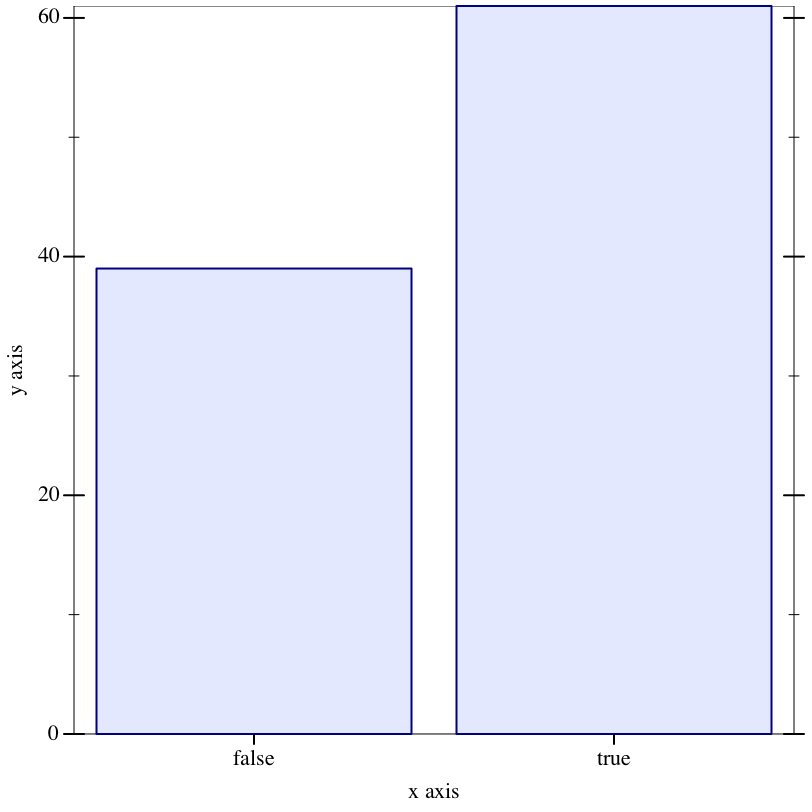> (hist (repeat s-or 1000))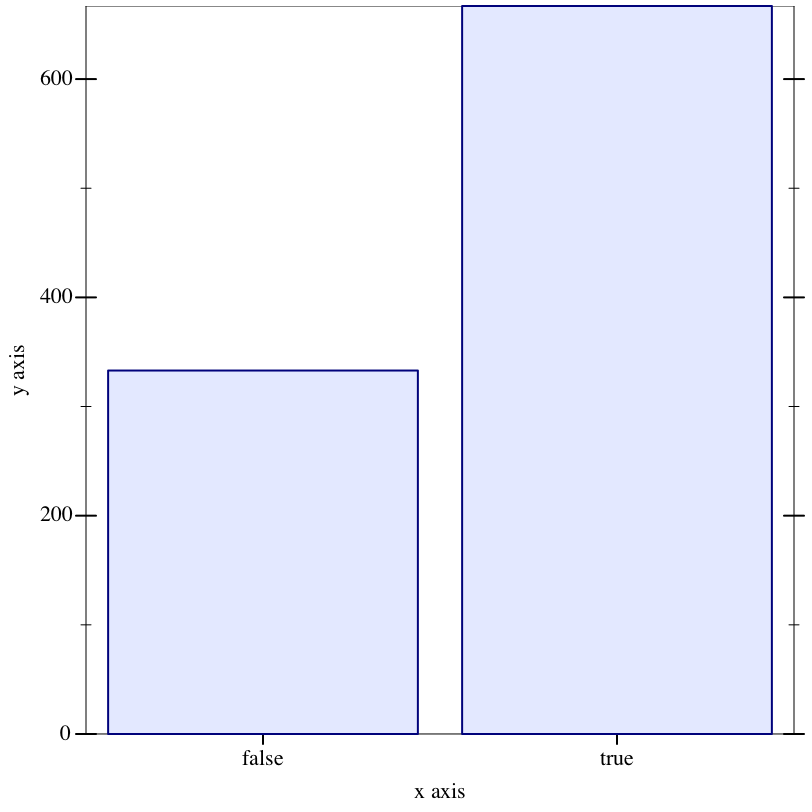7

7

> (hist (repeat rs-count-heads 100))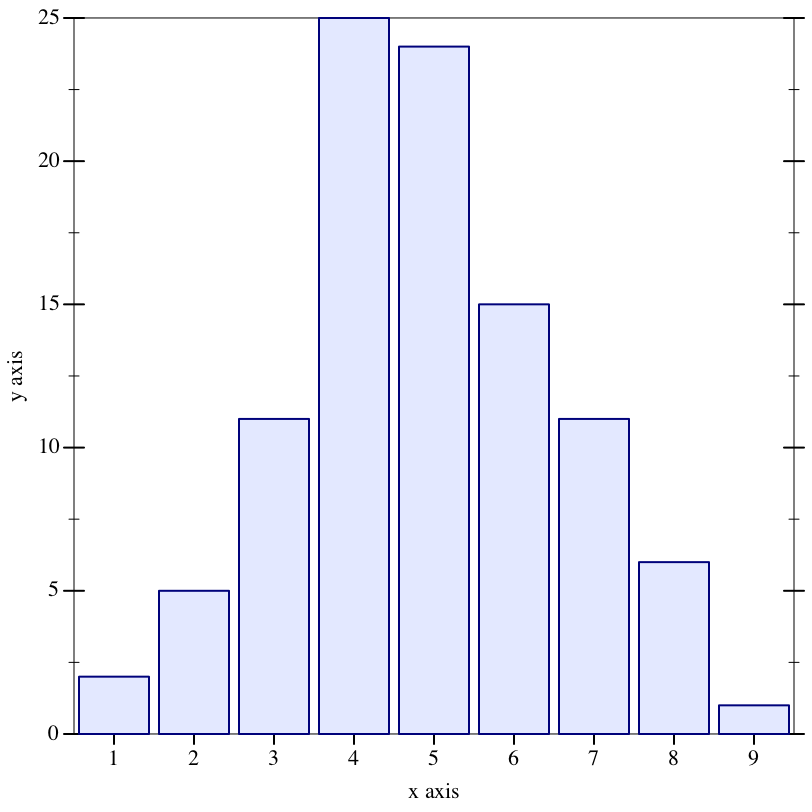syntax(importance-sampler def/expr ... result-expr)
Like rejection-sampler, but returns a weighted sampler that uses weights to represent the quality of a particular sample given the observations in the program. Thus unlike a rejection sampler, an importance sampler can handle observations (observe-sample) on continuous random variables.

#### 5.3Metropolis-Hastings Sampler and Transitions

Metropolis-Hastings (MH) is an algorithm framework for producing a correlated sequence of samples where each sample is based on the previous. The algorithm is parameterized by the mechanism for proposing a new state given the previous state; given a proposal, the MH algorithm accepts or rejects it based on how the proposal was generated and the relative likelihood of the proposed state.

The mh-sampler form implements the Metropolis-Hastings framework, and the proposal mechanisms are implemented by a variety of MH transition types.

syntax

(mh-sampler maybe-transition def/expr ... result-expr)

maybe-transition =
| #:transition transition-expr
Returns a sampler that produces samples using a variant of Metropolis-Hastings.

The transition-expr determines the mechanism used to propose new states. If absent, (single-site) is used.

Examples:

 > (define mh-or (mh-sampler (define A (flip)) (define B (flip)) (observe/fail (or A B)) A))
> (hist (repeat mh-or 100))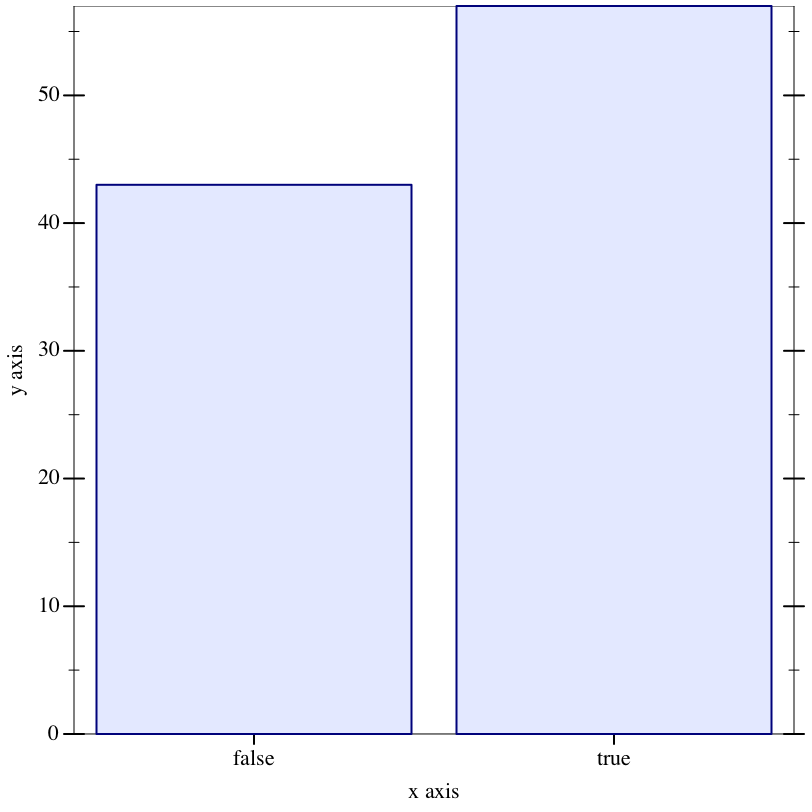> (define mh-n-flips (mh-sampler (count-heads 10)))
 > (parameterize ((verbose? #t)) (mh-n-flips))
 # Starting transition (rerun-mh-transition%) #   NEW (bernoulli-dist 1/2): (1 3 46 83) = 1 #   NEW (bernoulli-dist 1/2): (1 3 46 47 83) = 1 #   NEW (bernoulli-dist 1/2): (1 3 46 47 47 83) = 1 #   NEW (bernoulli-dist 1/2): (1 3 46 47 47 47 83) = 0 #   NEW (bernoulli-dist 1/2): (1 3 46 47 47 47 47 83) = 0 #   NEW (bernoulli-dist 1/2): (1 3 46 47 47 47 47 47 83) = 1 #   NEW (bernoulli-dist 1/2): (1 3 46 47 47 47 47 47 47 83) = 0 #   NEW (bernoulli-dist 1/2): (1 3 46 47 47 47 47 47 47 47 83) = 1 #   NEW (bernoulli-dist 1/2): (1 3 46 47 47 47 47 47 47 47 47 83) = 1 #   NEW (bernoulli-dist 1/2): (1 3 46 47 47 47 47 47 47 47 47 47 83) = 0 # accept threshold = +inf.0 (density dimension +inf.0 -> 0) # Accepted MH step with 0.2766589954367539

6

 > (parameterize ((verbose? #t)) (mh-n-flips))
 # Starting transition (single-site-mh-transition%) # key to change = (1 3 46 47 47 47 83) # DRIFTED from 0 to 1 # PROPOSED from 0 to 1 #   R/F = 1 #   REUSED (bernoulli-dist 1/2): (1 3 46 83) = 1 #   REUSED (bernoulli-dist 1/2): (1 3 46 47 83) = 1 #   REUSED (bernoulli-dist 1/2): (1 3 46 47 47 83) = 1 #   PERTURBED (bernoulli-dist 1/2): (1 3 46 47 47 47 83) = 1 #   REUSED (bernoulli-dist 1/2): (1 3 46 47 47 47 47 83) = 0 #   REUSED (bernoulli-dist 1/2): (1 3 46 47 47 47 47 47 83) = 1 #   REUSED (bernoulli-dist 1/2): (1 3 46 47 47 47 47 47 47 83) = 0 #   REUSED (bernoulli-dist 1/2): (1 3 46 47 47 47 47 47 47 47 83) = 1 #   REUSED (bernoulli-dist 1/2): (1 3 46 47 47 47 47 47 47 47 47 83) = 1 #   REUSED (bernoulli-dist 1/2): (1 3 46 47 47 47 47 47 47 47 47 47 83) = 0 # accept threshold = 1.0 (density dimension 0 -> 0) # Accepted MH step with 0.7509922846700986

7

> (hist (repeat mh-n-flips 100))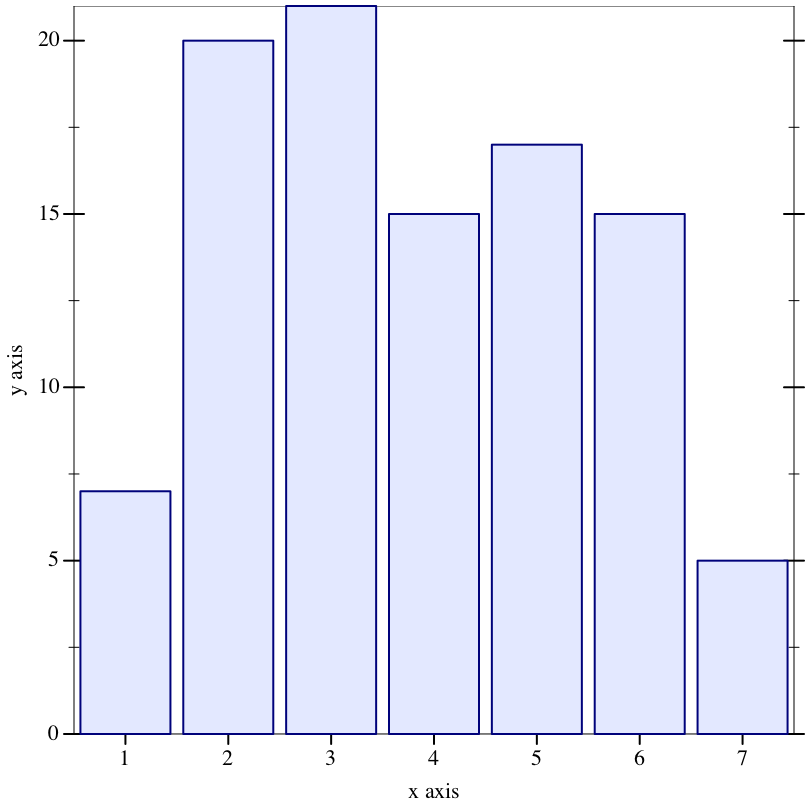> (hist (repeat mh-n-flips 2000))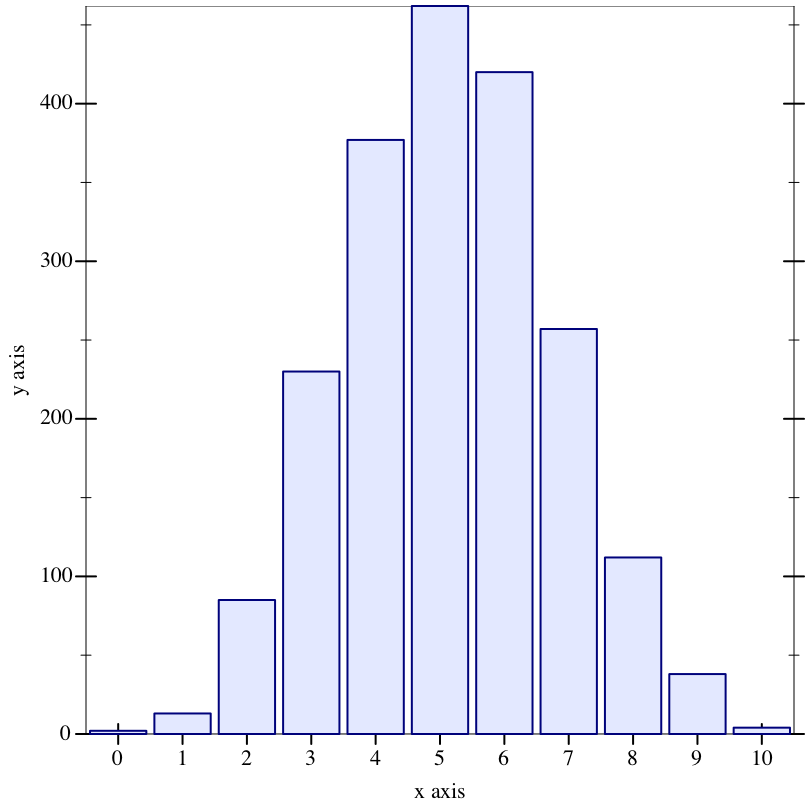##### 5.3.1Metropolis-Hastings Transitions

 procedure v : any/c
Returns #t if v represents a MH transition, #f otherwise.

procedure

(single-site [proposal #:zone zone-pattern])  mh-transition?

 proposal : (or/c proposal? (-> proposal?)) = (default-proposal)
zone-pattern : any/c = #f
A transition that proposes a new state by randomly (uniformly) selecting a single random choice in any zone matching zone-pattern and perturbing it according to proposal (see Metropolis-Hastings Proposals).

A zone pattern matches a zone if the two values are equal? or if the zone pattern is #f.

procedure

(multi-site [proposal #:zone zone-pattern])  mh-transition?

 proposal : (or/c proposal? (-> proposal?)) = (default-proposal)
zone-pattern : any/c = #f
A transition that proposes a new state by perturbing all random choices in all zones matching zone-pattern.

procedure

 (slice [ #:scale scale-factor #:zone zone-pattern]) → mh-transition?
scale-factor : (>/c 0) = 1
zone-pattern : any/c = #f
A transition that picks a new state via slice sampling on a single random choice selected randomly from any zone matching zone-pattern.

The scale-factor argument controls the width parameter used to find the slice bounds.

 procedure(enumerative-gibbs [#:zone zone-pattern]) → mh-transition? zone-pattern : any/c = #f
A transition that chooses a single random choice from a zone matching zone-pattern and resamples it from its full conditional probability distribution given the values of all of the other choices in the program. The distribution of the choice to be perturbed must be finite; otherwise, an error is raised. The choice to be perturbed must be non-structuralthat is, its value must not determine whether subsequent choices are made or not—otherwise, an error is raised.

Note: unlike traditional Gibbs sampling, this transition picks a choice at random rather than perturbing all choices round-robin.

 procedure(cycle tx ...+) → mh-transition? tx : mh-transition?
Returns a transition that uses an endless cycle of the txs to produce samples.

 procedure(mixture txs [weights]) → mh-transition? txs : (listof mh-transition?) weights : (listof (>/c 0)) = (map (lambda (tx) 1) txs)
Returns a transition that randomly selects a transition from txs, weighted by weights, on each step.

 procedure
Returns a transition that just reruns the program with the same state as the previous execution.

##### 5.3.2Metropolis-Hastings Proposals

The single-site and multi-site transitions are parameterized by the proposal distribution used to perturb a single random choice.

 procedure v : any/c
Returns #t if v is a proposal object, #f otherwise.

 procedure
Returns a proposal that chooses the new value of a variable by simply resampling from the variable’s prior distribution.

 procedure
Returns a proposal that chooses the new value of a variable by choosing a value near the current value, with an adaptive variance.

 parameter (default-proposal proposal) → void? proposal : (or/c proposal? (-> proposal?))
Parameter for the default proposal used by MH transitions such as single-site and multi-site. If the value of the parameter is a function, it is applied when the transition is created to get an actual proposal.

The initial value is proposal:drift.

##### 5.3.3Zones: Identifying Random Choices

Random choices made during the execution of a model can be grouped into zones using the with-zone form. Specialized transitions can then be assigned to choices based on their membership in zones.

 syntax(with-zone zone-expr body ...+)
Associate each random choice in the dynamic extent of the evaluation of the body forms with the zone produced by zone-expr. Any value except #f can identity a zone.

#### 5.4Particle Filters

A particle set consists of a collection of particles, each of which contains a current state estimate and a likelihood weight. Particle sets are updated via a (stochastic) state transformer that produces a new state for each particle. Observations performed by the state transformer adjust the particles’ weights.

 procedure v : any/c
Returns #t if v is a particle set, #f otherwise.

 procedure(make-particles n init-state) → particles? n : exact-nonnegative-integer? init-state : any/c
Returns a particle set with n particles, each with state init-state and weight 1.

 procedure ps : particles?
Returns the number of particles in ps.

 procedure(particles-update ps update-state) → particles? ps : particles? update-state : (-> any/c any/c)
Produces a new particle set where each particle corresponds to a particle in ps, where each new particle’s state is the result of applying update-state to the old state. Each new particle’s weight is the old particle’s weight adjusted by observations performed by update-state.

 procedure(particles-score ps score-state) → particles? ps : particles? score-state : (-> any/c any)
Like particles-update, but the result of the score-state function is ignored and the state of each particle is unchanged. Observations performed by score-state still affect the new particles’ weights.

Equivalent to (particles-update ps (lambda (st) (score-state st) st)).

 procedure(particles-resample ps [n #:alg algorithm]) → particles? ps : particles? n : exact-nonnegative-integer? = (particles-count ps) algorithm : (or/c 'multinomial 'residual #f) = 'multinomial
Produces a new particle set by resampling particles from ps. Every particle in the new particle set has weight 1. See also resample.

 procedure ps : particles?
 procedure ps : particles?
Returns an estimate of the effective sample size and its ratio to the number of particles, respectively.

 procedure → (vectorof (cons/c any/c (>/c 0))) ps : particles?
Returns a vector of the particle states and weights from ps. Particles with zero weight are omitted, so the length of the vector may be less than (particle-count ps).

 procedure ps : particles?
Returns a vector of the particle states from ps, regardless of weight (except that particles with zero weight are omitted).

In general, it is only sensible to call this function when the weights are known to be equal, such as after calling particles-resample.

 procedure ps : particles?
Produces a sequence where each step produces two values: the particle state and its weight. Particles with empty weights are omitted from the sequence.

#### 5.5Enumeration Solver

syntax

 (enumerate maybe-limit maybe-normalize def/expr ... result-expr)

maybe-limit =
| #:limit limit-expr

maybe-normalize =
| #:normalize? normalize?-expr

 limit-expr : (or/c #f (>=/c 0))
 normalize?-expr : any/c
Returns a discrete distribution of the form (discrete-dist [value prob] ...), where value is a value produced by result-expr and prob is its normalized probability given condition-expr and the observations in the program. If normalize?-expr is given and evaluates to #f, then the probability is unnormalized instead.

The enumerate form works by exploring all possibilities using the technique described in [EPP]. If limit-expr is absent or evaluates to #f, then exploration ceases only when all paths have been explored; if any path is infinite, then enumerate fails to terminate. If limit-expr is given and evaluates to a probability limit, then exploration ceases when the error of the normalized result distribution would be less than limitthat is, when the unexplored paths have probability less than limit times the sum of the probabilities of the paths accepted so far.

Only discrete and integer-valued distributions can be sampled with enumerate, and infinite-range distributions (such as geometric-dist) and infinitely-deep recursive functions (such as geometric) require the use of #:limit for termination.

Example:

 > (enumerate (define A (flip)) (define B (flip)) (observe/fail (or A B)) A)

(discrete-dist [#f 1/3] [#t 2/3])

Use #:limit to control when exploration should be cut off.

 > (enumerate #:limit 1e-06 (geom))
 (discrete-dist [0 524288/1048575] [1 262144/1048575] [2 131072/1048575] [3 65536/1048575] [4 32768/1048575] [5 16384/1048575] [6 8192/1048575] [7 4096/1048575] [8 2048/1048575] [9 1024/1048575] [10 512/1048575] [11 256/1048575] [12 128/1048575] [13 64/1048575] [14 32/1048575] [15 16/1048575] [16 8/1048575] [17 4/1048575] [18 2/1048575] [19 1/1048575])

Use #:normalize? #f to get the result probabilities without normalizing by the acceptance rate:

 > (enumerate #:normalize? #f (define (drop-coin?) (flip 0.9)) (define (drunk-flips n) (cond [(zero? n) #t] [(drop-coin?) 'failed] [else (and (flip) (drunk-flips (sub1 n)))])) (define A (drunk-flips 10)) (observe/fail (not (eq? A 'failed))) (eq? A #t))

(discrete-measure [#f 1.0] [#t 1.855468750000192e-12])